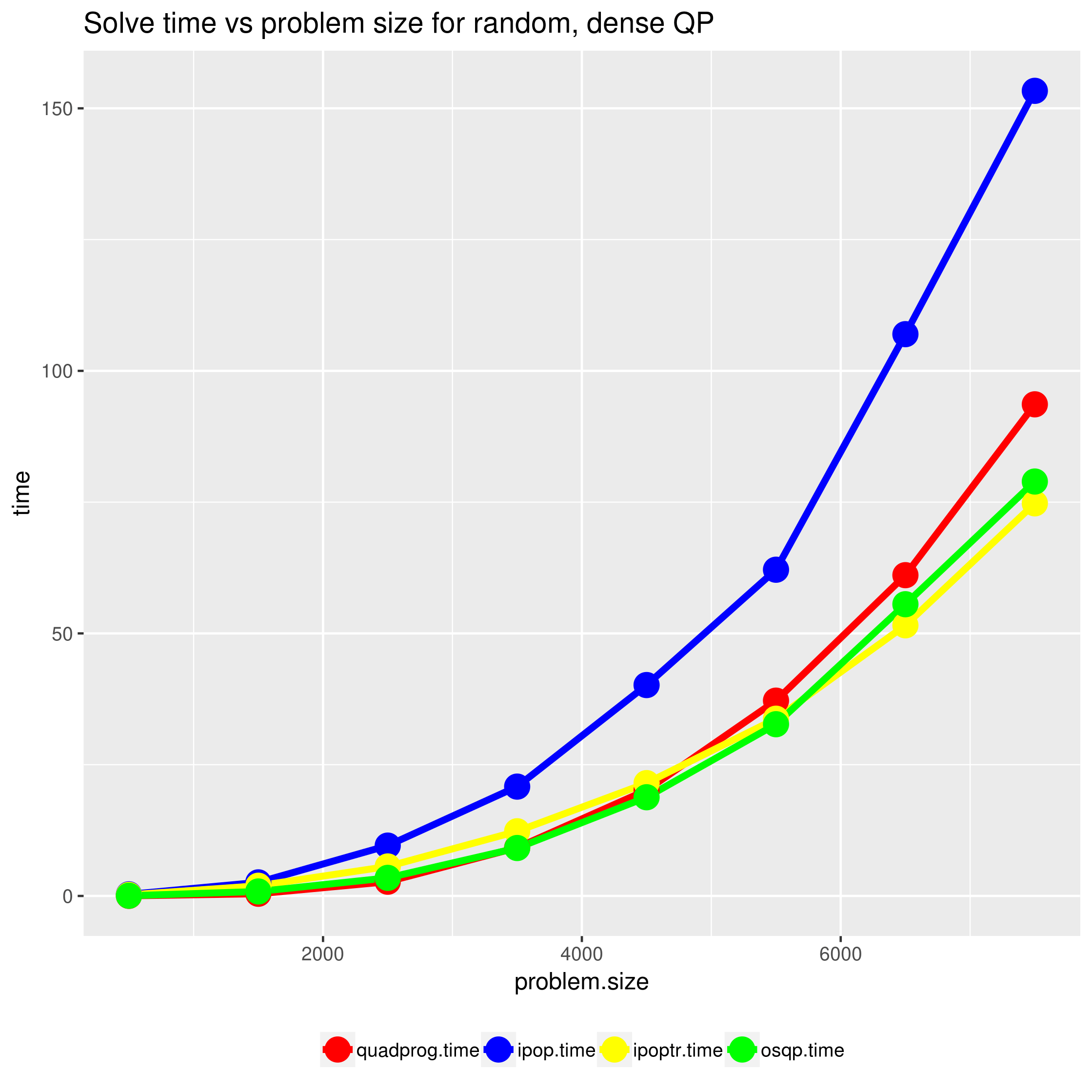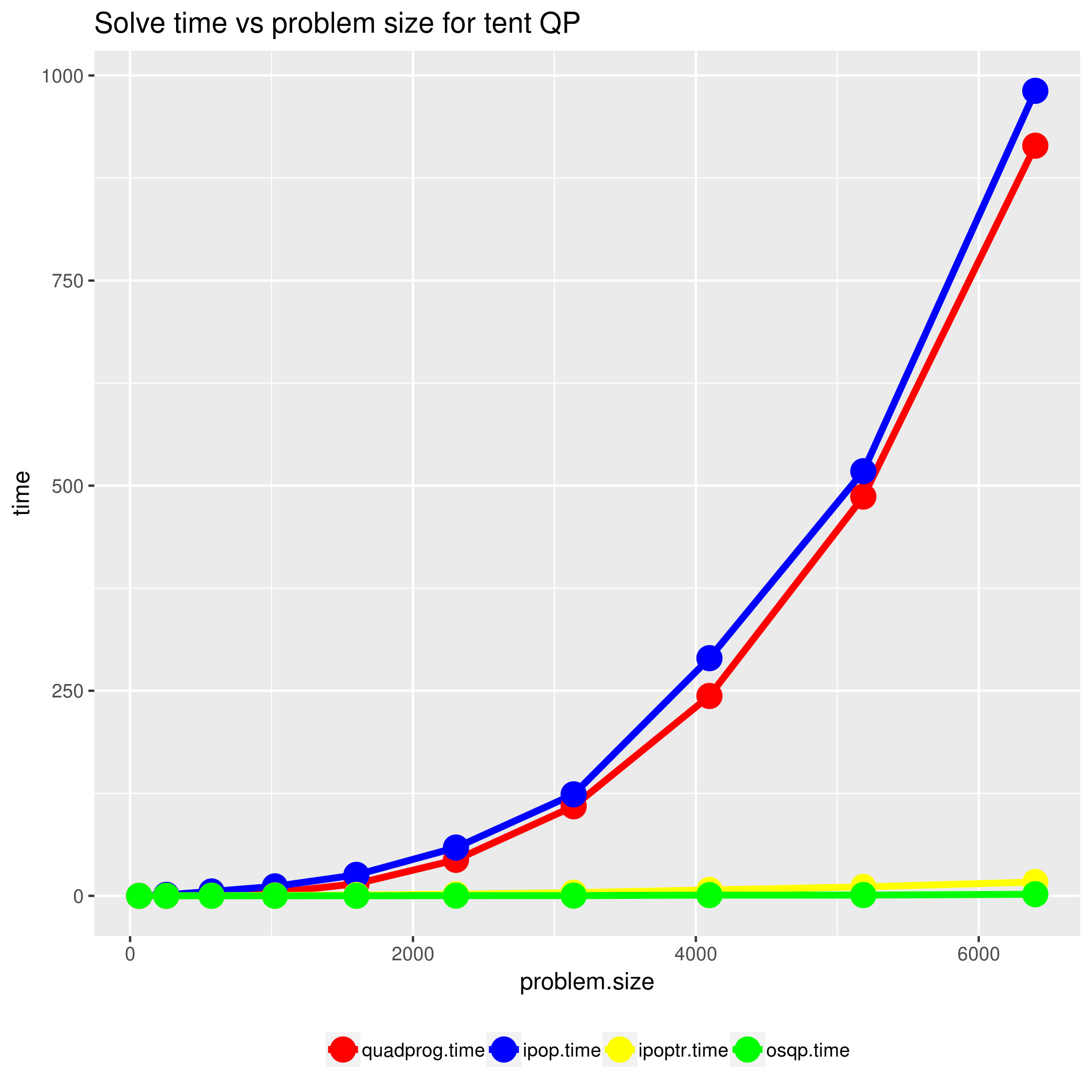# Sparse quadratic programming with osqp

In the past, I wrote frequently about quadratic programming especially in R, for example here and here. It’s been a while and at least one great new library has emerged since my last post on quadratic programming — OSQP. OSQP introduces a new technique called operator splitting which offers significant performance improvements over standard interior point algorithms on large, sparse QPs. OSQP is an open source C library, with interfaces for many languages, including R!

Let’s take this new library for a spin and present some simple benchmarks.

## Getting started

The R OSPQ interface is available in CRAN and installation is simple:

install.packages("ospq")


OSPQ solves quadratic programs of the form:
\begin{aligned} \underset{\alpha \in \mathbb{R}^n}{\text{Minimize}}: \qquad & q^Tx + \frac{1}{2}x^T P x \\ \text{Subject to:} \qquad & l \leq Ax \leq u \end{aligned}
where $P$ is positive semi-definite.

The quadprog package is the gold-standard for solving quadratic programs in R. Let’s see how we’d solve quadprog’s documentation example with OSPQ:

Note that quadprog uses a slightly obtuse specification of the functional form for the QP that comes directly out of the original paper on which it is based. OSQP uses the more standard specification from above. To solve a quadprog form QP in OSQP we just need to negate the dvec and transpose Amat. More substantively, two very important differences between OSQP and quadprog are:

• OSPQ can handle sparse system matrices while quadprog cannot. This is quite important as many practical problems, especially in physics, will exhibit sparsity in $P$ or $A$ or both.
• quadprog can handle only positive definite matrices $P$ while OSPQ can handle positive semi-definite $P$. This distinction is important for problems like SVM.

## A Benchmark Problem

I benchmarked OSQP on two problems, a random, dense quadratic program and the circus tent problem I’ve used in previous posts.

I’ve packaged my benchmarking code in this Github repo.
Here are the results:In these experiments, OSQP and ipoptr fair quite similarly and both are significantly faster than quadprog (FORTRAN) and kernlab’s ipop (pure R). Though OSQP didn’t appear to be significantly faster in these trials, it offers the following significant advantages over ipoptr:

• It is much easier to install OSQP. ipoptr has a number of system dependencies and is quite tricky to get running.
• As a general optimization library, ipoptr has a much more generic interface specification and requires a computation of the Jacobian, the Hessian, and several other inputs. By contrast, OSQP needs only the system matrices for the QP.

Bottom line, if you need to solve large QPs, OSQP is the new way to go!